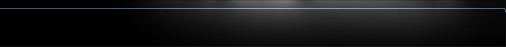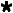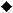//HOME User Manual Glossary Why HIMIPref™ Updates & Notices Contact Us# Yields

## Yield Measure Properties

It is not enough to determine the yield for a fixed-income instrument. To implement a rational investment programme, one must also have some idea of how the instrument will behave in a dynamic interest rate environment. The most popular and useful measures of this quality are Macaulay Duration and Modified Duration, the latter value representing the first derivative of the plot of Price vs. Yield. The second derivative of such a graph is referred to as convexity and is, for instruments without embedded options, positive.

Although convexity is of limited quantitative utility in traditional fixed income analysis (expressions such as "The benter the better!" notwithstanding), convexity can represent a useful qualitative tool in analyzing and describing fixed income portfolios. A positive convexity is generally a good thing; as market yields change, the sensitivity of the instrument will increase with increasing price and decrease with decreasing price, providing at least a certain amount of comfort to portfolio managers. With a negative-convexity instrument, however, the opposite is true, with the effect that when the market moves against a position the deterioration will accelerate with the losses.

The HIMIPref™ analytical system incorporates various measures subordinate to Yield in its examination of individual instruments and portfolios these are referenced in the following table and explained below:

 Yield Measures and Dependent Properties Yield Measure Macaulay Duration Modified Duration Pseudo-Modified Duration Pseudo-Convexity Current YieldYield-to-WorstPorfolio YieldGraphGraphGraph Cost YieldCurve YieldKEY:Calculated and reportedThis value is calculated, but not explicitly reported.Not calculated

## Usefulness of the Dependent Properties

### Macaulay Duration

This value is calculated for each sequence of cash flows in the same manner as is standard in fixed income calculation (and a precise derivation of the result is presented in a durationCalculationBox). The value reported for "Portfolio Yield" is a probability-weighted average of the values found for each component of the "Portfolio".

### Modified Duration

This value is also calculated in the standard manner but due to the limited utility of the value, it is only calculated for the Yield-to-Worst element. Note that HIMIPref™ defines the "Yield-to-Worst" scenario as being that element of the "Portfolio Yield" portfolio which has the lowest yield - this number may not always be precisely the same as that found by an examination of the embedded options to find the absolutely worst sequence of events.

### Pseudo-Modified Duration

This is a measure developed by Hymas Investment Management Inc. specifically for use with HIMIPref™. In an environment with embedded options, there is no closed-form equation possible which will legitimately provide a first derivative of the Price/Yield curve, so pseudo-modified-duration is calculated as an approximation. Three points on the Price/Yield curve are selected - one representing the market price and others 1% above and below this price. Yields are calculated ab initio for these three points and pseudo-modified-duration is calculated according to the slope of a line connecting the two outermost-points.

A separate determination of Pseudo-Modified-Duration is made using each of Yield-to-Worst, Portfolio Yield and Cost Yield as the basis for calculation. This calculation is summarized, with supporting data, in the pseudoModifiedDurationCalculationBox (Image | Glossary)

### Pseudo-Convexity

This proprietary measure has been developed for HIMIPref™ to provide information equivalent to that usually provided by "convexity" in an option-free fixed income environment. Similarly to the calculation of "Pseudo-Modified-Duration", three prices are selected, but in this case it is Pseudo-Modified-Duration that is calculated for each of these three points - the slope of the line between the two outermost points is considered to be the "Pseudo-Convexity".

A separate determination of Pseudo-Convexity is made using each of Yield-to-Worst, Portfolio Yield and Cost Yield as the basis for calculation. This calculation is summarized, with supporting data, in the pseudoConvexityCalculationBox (Image | Glossary)